## Function to visualise input data matrix using advanced heatmap

### Description

`visHeatmapAdv` is supposed to visualise input data matrix using advanced heatmap. It allows for adding multiple sidecolors in both columns and rows. Besides, the sidecolor can be automatically added via cutting histogram into groups. Note: this heatmap displays matrix in a top-to-bottom direction

### Usage

```visHeatmapAdv(data, scale = c("none", "row", "column"), Rowv = TRUE, Colv = TRUE,
dendrogram = c("both", "row", "column", "none"), dist.metric = c("euclidean",
"pearson", "spearman", "kendall", "manhattan", "cos", "mi"), linkage.method = c("complete",
"ward", "single", "average", "mcquitty", "median", "centroid"), colormap = c("bwr",
"jet", "gbr", "wyr", "br", "yr", "rainbow", "wb"), ncolors = 64, zlim = NULL,
RowSideColors = NULL, row.cutree = NULL, row.colormap = c("jet"), ColSideColors = NULL,
column.cutree = NULL, column.colormap = c("jet"), ...)```

### Arguments

data
an input gene-sample data matrix used for heatmap
scale
a character indicating when the input matrix should be centered and scaled. It can be one of "none" (no scaling), "row" (being scaled in the row direction), "column" (being scaled in the column direction)
Rowv
determines if and how the row dendrogram should be reordered. By default, it is TRUE, which implies dendrogram is computed and reordered based on row means. If NULL or FALSE, then no dendrogram is computed and no reordering is done. If a dendrogram, then it is used "as-is", ie without any reordering. If a vector of integers, then dendrogram is computed and reordered based on the order of the vector
Colv
determines if and how the column dendrogram should be reordered. Has the options as the Rowv argument above and additionally when x is a square matrix, Colv = "Rowv" means that columns should be treated identically to the rows
dendrogram
character string indicating whether to draw 'none', 'row', 'column' or 'both' dendrograms. Defaults to 'both'. However, if Rowv (or Colv) is FALSE or NULL and dendrogram is 'both', then a warning is issued and Rowv (or Colv) arguments are honoured
dist.metric
distance metric used to calculate the distance metric between columns (or rows). It can be one of "none" (i.e. no dendrogram between rows), "pearson", "spearman", "kendall", "euclidean", "manhattan", "cos" and "mi". See details at http://suprahex.r-forge.r-project.org/sDistance.html
the agglomeration method used to cluster/linkages columns (or rows). This should be one of "ward", "single", "complete", "average", "mcquitty", "median" or "centroid". See 'Note' below for details
colormap
short name for the colormap. It can be one of "jet" (jet colormap), "bwr" (blue-white-red colormap), "gbr" (green-black-red colormap), "wyr" (white-yellow-red colormap), "br" (black-red colormap), "yr" (yellow-red colormap), "wb" (white-black colormap), and "rainbow" (rainbow colormap, that is, red-yellow-green-cyan-blue-magenta). Alternatively, any hyphen-separated HTML color names, e.g. "blue-black-yellow", "royalblue-white-sandybrown", "darkgreen-white-darkviolet". A list of standard color names can be found in http://html-color-codes.info/color-names
ncolors
the number of colors specified over the colormap
zlim
the minimum and maximum z/patttern values for which colors should be plotted, defaulting to the range of the finite values of z. Each of the given colors will be used to color an equispaced interval of this range. The midpoints of the intervals cover the range, so that values just outside the range will be plotted
RowSideColors
NULL or a matrix of "numRowsidebars" X nrow(x), where "numRowsidebars" stands for the number of sidebars annotating rows of x. This matrix contains the color names for vertical sidebars. By default, it sets to NULL. In this case, sidebars in rows can still be enabled by cutting the row dendrogram into several clusters (see the next two parameters)
row.cutree
an integer scalar specifying the desired number of groups being cut from the row dendrogram. Note, this optional is only enabled when the ColSideColors is NULL
row.colormap
short name for the colormap to color-code the row groups (i.e. sidebar colors used to annotate the rows)
ColSideColors
NULL or a matrix of ncol(x) X "numColsidebars", where "numColsidebars" stands for the number of sidebars annotating the columns of x. This matrix contains the color names for horizontal sidebars. By default, it sets to NULL. In this case, sidebars in columns can still be enabled by cutting the column dendrogram into several clusters (see the next two parameters)
column.cutree
an integer scalar specifying the desired number of groups being cut from the column dendrogram. Note, this optional is only enabled when the column dengrogram is built
column.colormap
short name for the colormap to color-code the column groups (i.e. sidebar colors used to annotate the columns)
...
additional graphic parameters. For most parameters, please refer to https://www.rdocumentation.org/packages/gplots/topics/heatmap.2. For example, the parameters "srtRow" and "srtCol" to control the angle of row/column labels (in degrees from horizontal: 45 degrees for the column, 0 degrees for the row, by default), i.e. string rotation. The parameters "offsetRow" and "offsetCol" to indicate the number of character-width spaces to place between row/column labels and the edge of the plotting region. Unique to this function, there are two parameters "RowSideWidth" and RowSideLabelLocation, to respectively indicate the fraction of the row side width and the location (either bottom or top) of the row side labelling; the other two parameters "ColSideHeight" and "ColSideLabelLocation" for the column side height and the location (either left or right) of the column side labelling; and two parameters "RowSideBox" and "ColSideBox" to indicate whether there are boxes outside.

invisible

### Note

• "ward": Ward's minimum variance method aims at finding compact, spherical clusters
• "single": The single linkage method (which is closely related to the minimal spanning tree) adopts a 'friends of friends' clustering strategy
• "complete": The complete linkage method finds similar clusters
• "average","mcquitty","median","centroid": These methods can be regarded as aiming for clusters with characteristics somewhere between the single and complete link methods. Two methods "median" and "centroid" are not leading to a monotone distance measure, or equivalently the resulting dendrograms can have so called inversions (which are hard to interpret)

### Examples

```# 1) generate data with an iid matrix of 100 x 9
data <- cbind(matrix(rnorm(100*3,mean=0,sd=1), nrow=100, ncol=3),
matrix(rnorm(100*3,mean=0.5,sd=1), nrow=100, ncol=3),
matrix(rnorm(100*3,mean=-0.5,sd=1), nrow=100, ncol=3))
colnames(data) <-
c("S1_R1","S1_R2","S1_R3","S2_R1","S2_R2","S2_R3","S3_R1","S3_R2","S3_R3")

# 2) heatmap after clustering both rows and columns
# 2a) shown with row and column dendrograms
KeyValueName="log2(Ratio)",
lmat=rbind(c(4,3), c(2,1)), lhei=c(1,5), lwid=c(1,3))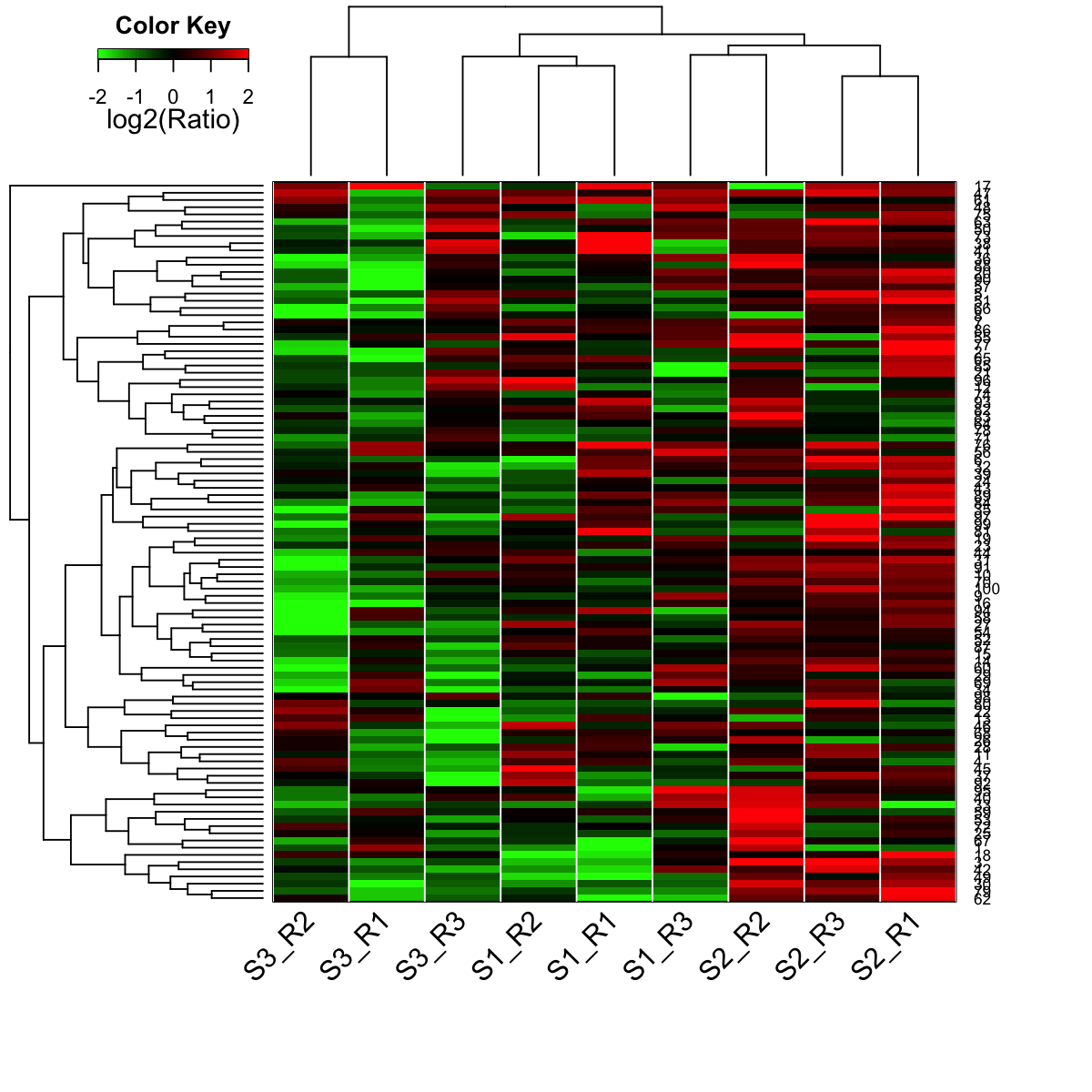# 2b) shown with row dendrogram only# 2c) shown with column dendrogram only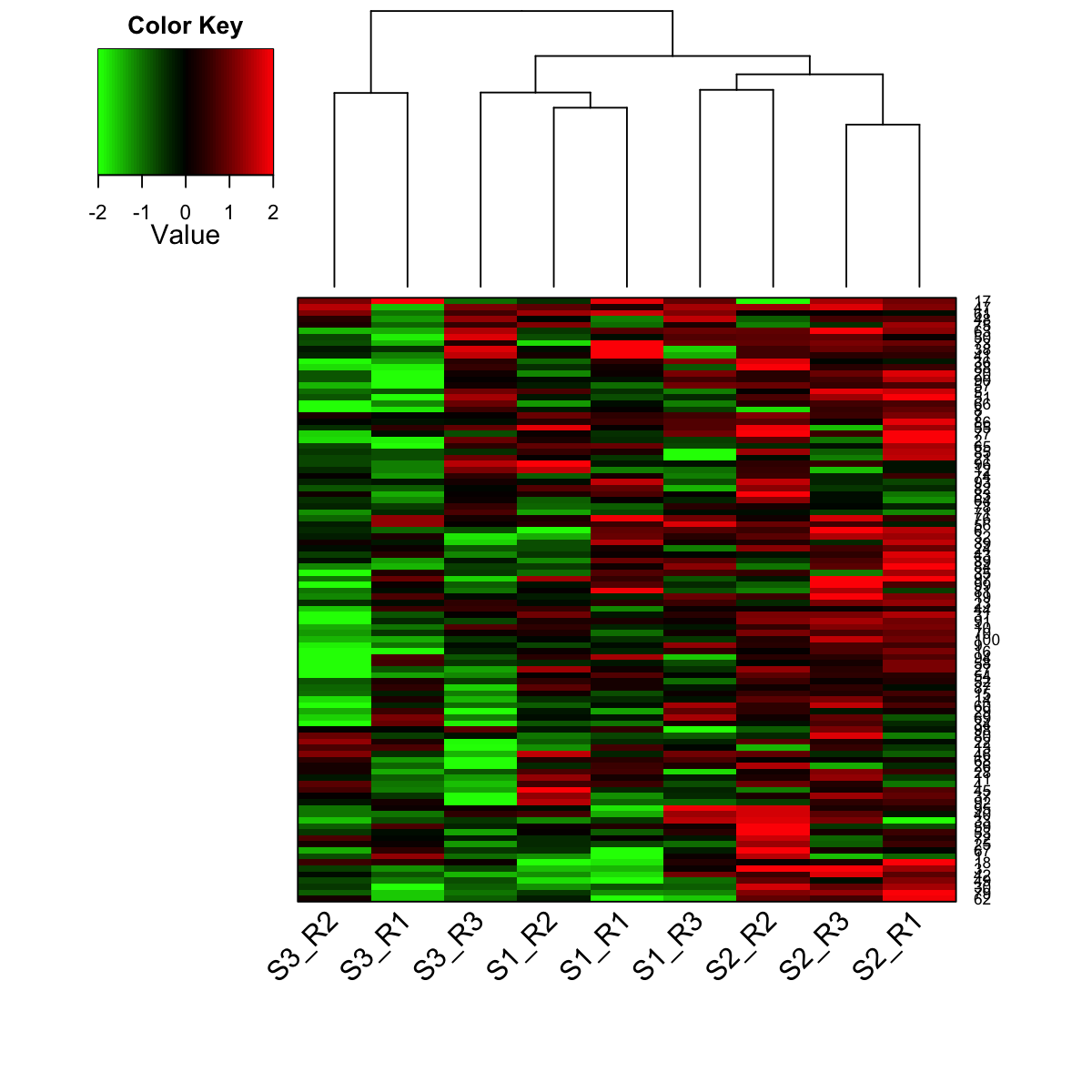# 3) heatmap after only clustering rows (with 2 color-coded groups)
row.cutree=2, row.colormap="jet", labRow=NA)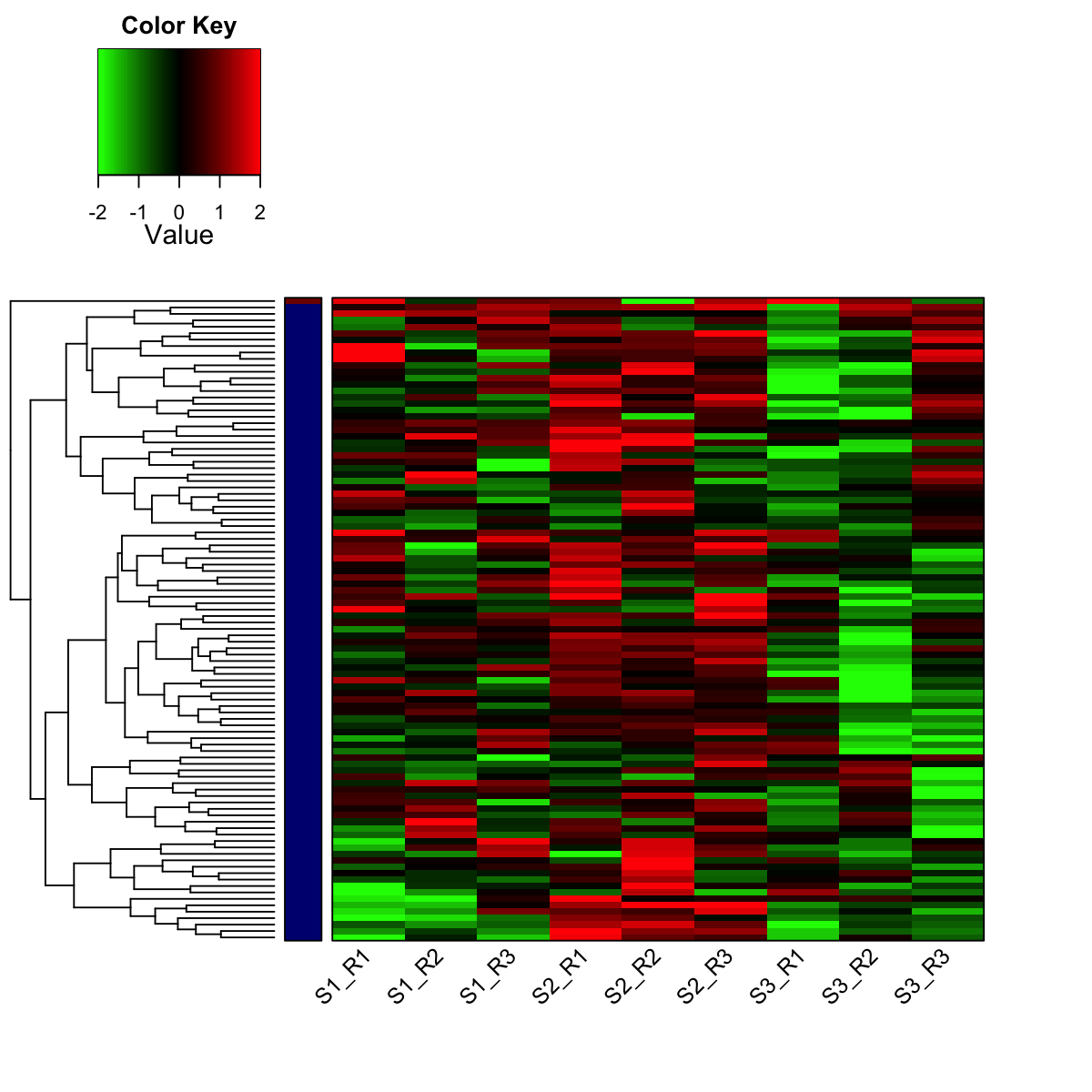# 4) prepare colors for the column sidebar
# color for stages (S1-S3)
stages <- sub("_.*","",colnames(data))
sta_lvs <- unique(stages)
sta_color <- visColormap(colormap="rainbow")(length(sta_lvs))
col_stages <- sapply(stages, function(x) sta_color[x==sta_lvs])
# color for replicates (R1-R3)
replicates <- sub(".*_","",colnames(data))
rep_lvs <- unique(replicates)
rep_color <- visColormap(colormap="rainbow")(length(rep_lvs))
col_replicates <- sapply(replicates, function(x) rep_color[x==rep_lvs])
# combine both color vectors
ColSideColors <- cbind(col_stages,col_replicates)
colnames(ColSideColors) <- c("Stages","Replicates")

# 5) heatmap without clustering on rows and columns but with the two sidebars in columns
zlim=c(-2,2),
density.info="density", tracecol="yellow", ColSideColors=ColSideColors,
ColSideHeight=0.5, ColSideLabelLocation="right")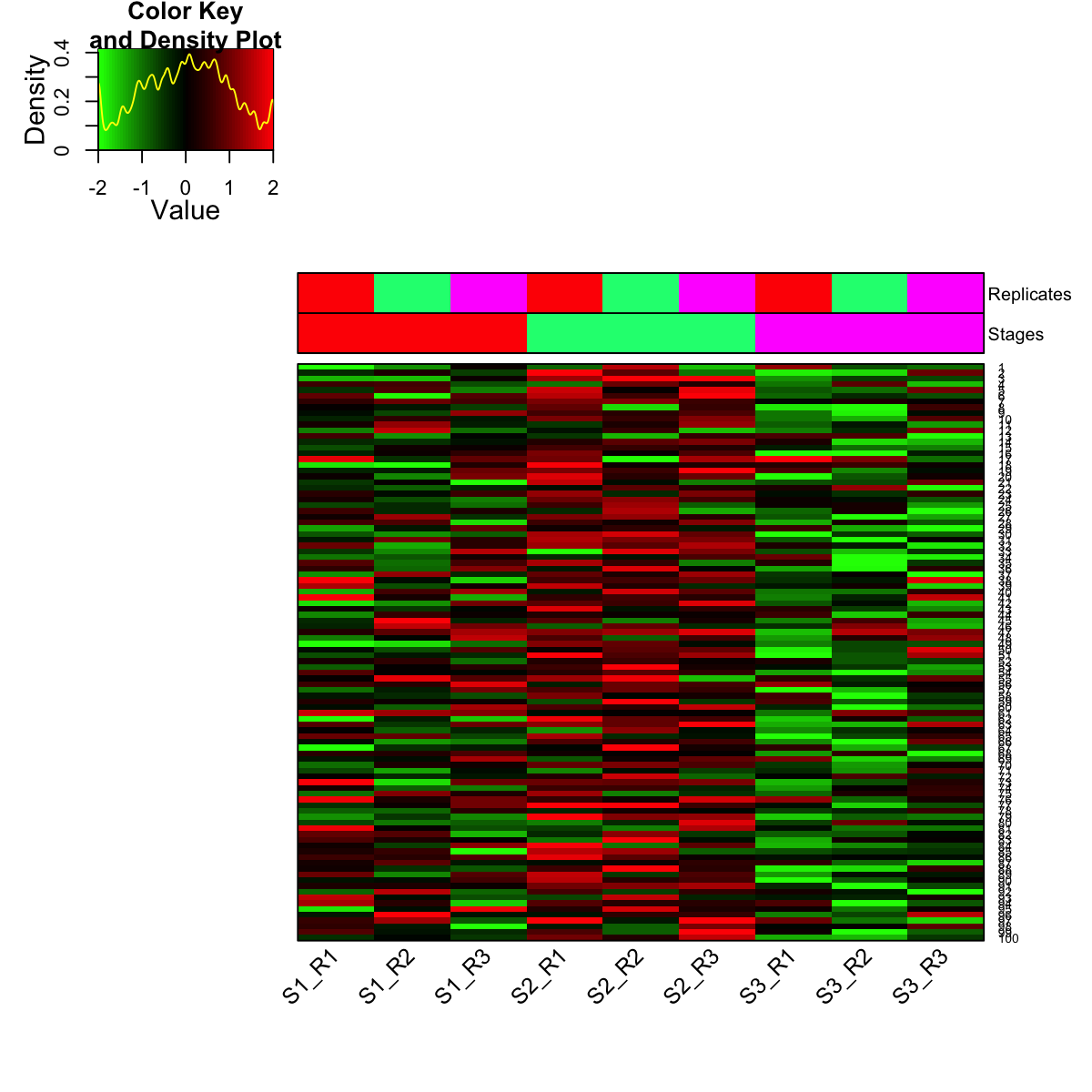# 6) legends
legend(0,0.8, legend=rep_lvs, col=rep_color, lty=1, lwd=5, cex=0.6,
box.col="transparent", horiz=FALSE)legend(0,0.6, legend=sta_lvs, col=sta_color, lty=1, lwd=5, cex=0.6,
box.col="transparent", horiz=FALSE)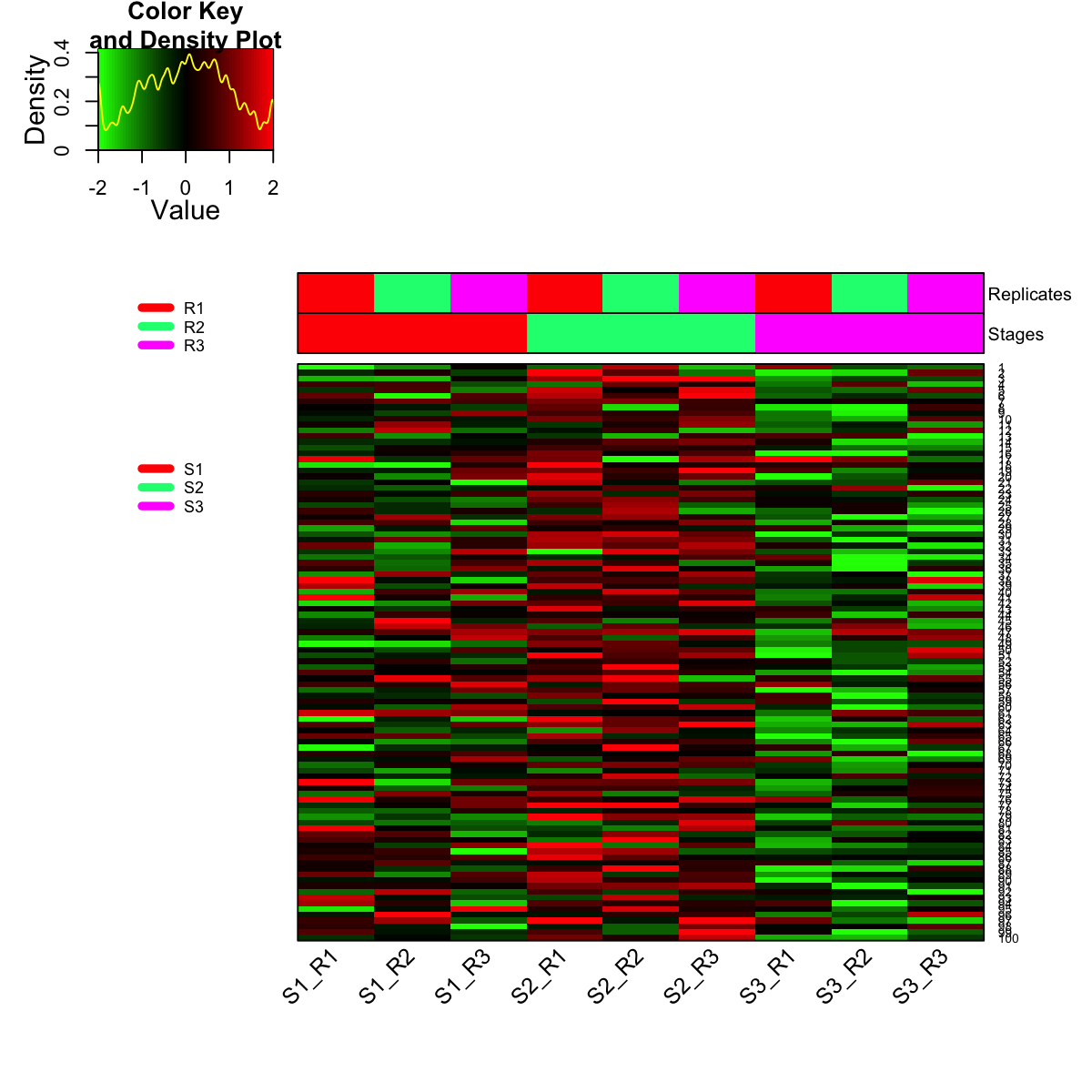```

## Source code

`visHeatmapAdv.r`

## Source man

`visHeatmapAdv.Rd` `visHeatmapAdv.pdf`

`visHeatmapAdv`